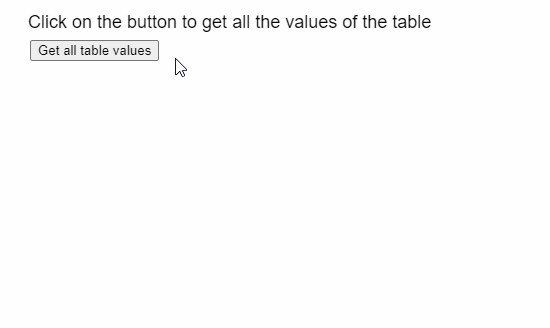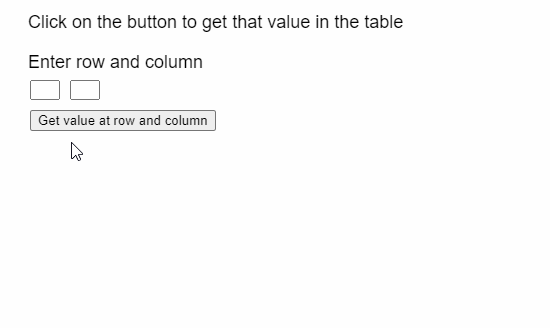# p5.Table get() Method

The get() method of p5.Table in p5.js is used to retrieve a value from the given row and column of the table. The row can be specified by the row ID and the column can be specified by its column ID or column name.

Syntax:

```get( row, column )
```

Parameters: This method accepts two parameters as mentioned above and described below:

• row: It is a number that denotes the row ID of the row.
• column: It is a String or Number that denotes the column name or ID of the column.

Return Value: This method returns the String or Number value retrieved from the given row and column of the table.

The examples below illustrate the get() method in p5.js:

Example 1:

## Javascript

 `function` `setup() { ` `  ``createCanvas(500, 300); ` `  ``textSize(18); ` ` `  `  ``text(``"Click on the button to get all "` `+  ` `       ``"the values of the table"``, 20, 20); ` ` `  `  ``setBtn = createButton(``"Get all table values"``); ` `  ``setBtn.position(30, 40); ` `  ``setBtn.mouseClicked(showTable); ` ` `  `  ``// Create the table ` `  ``table = ``new` `p5.Table(); ` ` `  `  ``setTableData(); ` `} ` ` `  `function` `setTableData() { ` ` `  `  ``// Add 5 columns and rows to the table ` `  ``for` `(let i = 0; i < 5; i++) { ` `    ``table.addColumn(``"Column "` `+ i); ` `    ``table.addRow(); ` `  ``} ` ` `  `  ``for` `(let r = 0; r < 5; r++) { ` `    ``for` `(let c = 0; c < 5; c++) { ` ` `  `      ``// Set the value at the given ` `      ``// column and row of the table ` `      ``table.set(r, c, r + ``" "` `+ c); ` `    ``} ` `  ``} ` `} ` ` `  `function` `showTable() { ` `  ``clear(); ` `  ``text(``"The values of the table are retrieved "` `+ ` `       ``"using the get() method"``, 20, 20); ` ` `  `  ``// Show all the columns present ` `  ``for` `(let c = 0; c < 5; c++) { ` `    ``text(table.columns, 20 + 100 * c, 80); ` `  ``} ` ` `  `  ``// Show all the rows currently ` `  ``// present in the table ` `  ``for` `(let r = 0; r < 5; r++) { ` `    ``for` `(let c = 0; c < 5; c++) { ` ` `  `      ``// Get the value at the given row ` `      ``// and column ` `      ``text(table.get(r, c), ` `          ``20 + 100 * c, ` `          ``100 + 20 * r); ` `    ``} ` `  ``} ` `} `

Output:Example 2:

## Javascript

 `function` `setup() { ` `  ``createCanvas(500, 300); ` `  ``textSize(18); ` ` `  `  ``text(``"Click on the button to get "` `+  ` `       ``"that value in the table"``, 20, 20); ` `   `  `  ``text(``"Enter row and column"``, 20, 60); ` ` `  `  ``rowInp = createInput(); ` `  ``rowInp.position(30, 80); ` `  ``rowInp.size(30, 20); ` ` `  `  ``colInp = createInput(); ` `  ``colInp.position(70, 80); ` `  ``colInp.size(30, 20); ` ` `  `  ``setBtn = ` `    ``createButton(``"Get value at row and column"``); ` `  ``setBtn.position(30, 110); ` `  ``setBtn.mouseClicked(getValueAt); ` ` `  `  ``// Create the table ` `  ``table = ``new` `p5.Table(); ` ` `  `  ``setTableData() ` `} ` ` `  `function` `setTableData() { ` ` `  `  ``// Add 5 columns and rows to the table ` `  ``for` `(let i = 0; i < 5; i++) { ` `    ``table.addColumn(``"Column "` `+ i); ` `    ``table.addRow(); ` `  ``} ` ` `  `  ``for` `(let r = 0; r < 5; r++) { ` `    ``for` `(let c = 0; c < 5; c++) { ` ` `  `      ``// Set the value at the given ` `      ``// column and row of the table ` `      ``table.set(r, c, r + ``" "` `+ c); ` `    ``} ` `  ``} ` `} ` ` `  `function` `getValueAt() { ` `  ``clear(); ` `  ``let rowToGet = int(rowInp.value()); ` `  ``let colToGet = int(colInp.value()); ` ` `  `  ``if` `(rowToGet < table.getRowCount() && ` `      ``colToGet < table.getColumnCount()) { ` `    ``text(``"The value at row "` `+ rowToGet +  ` `         ``" and column "` `+ colToGet + ``" is:"``, ` `         ``20, 160); ` ` `  `    ``// Get the value at the given row ` `    ``// and column ` `    ``text(table.get(rowToGet, colToGet), 20, 180); ` `  ``} ` `  ``else` `    ``text(``"Please enter correct row and "` `+ ` `    ``"column values"``, 20, 160); ` ` `  `  ``text(``"Click on the button to get that value "` `+  ` `       ``"in the table"``, 20, 20); ` `  ``text(``"Enter row and column"``, 20, 60); ` `}`

Output:Online editor: https://editor.p5js.org/My Personal Notes arrow_drop_upIm listening

If you like GeeksforGeeks and would like to contribute, you can also write an article using contribute.geeksforgeeks.org or mail your article to contribute@geeksforgeeks.org. See your article appearing on the GeeksforGeeks main page and help other Geeks.

Please Improve this article if you find anything incorrect by clicking on the "Improve Article" button below.

Article Tags :

Be the First to upvote.

Please write to us at contribute@geeksforgeeks.org to report any issue with the above content.